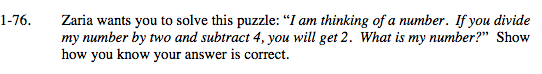### Home > MC2 > Chapter 1 > Lesson 1.2.3 > Problem1-76

1-76.Use the Guess and Check method.

Divide Zaria's number by 2, then subtract 4.

$\frac{?}{2} - 4 = 2$

Use the the eTool below to find the number that makes the equation true.
Click the link at right for the full version of the eTool: MC2 1-76 HW eTool